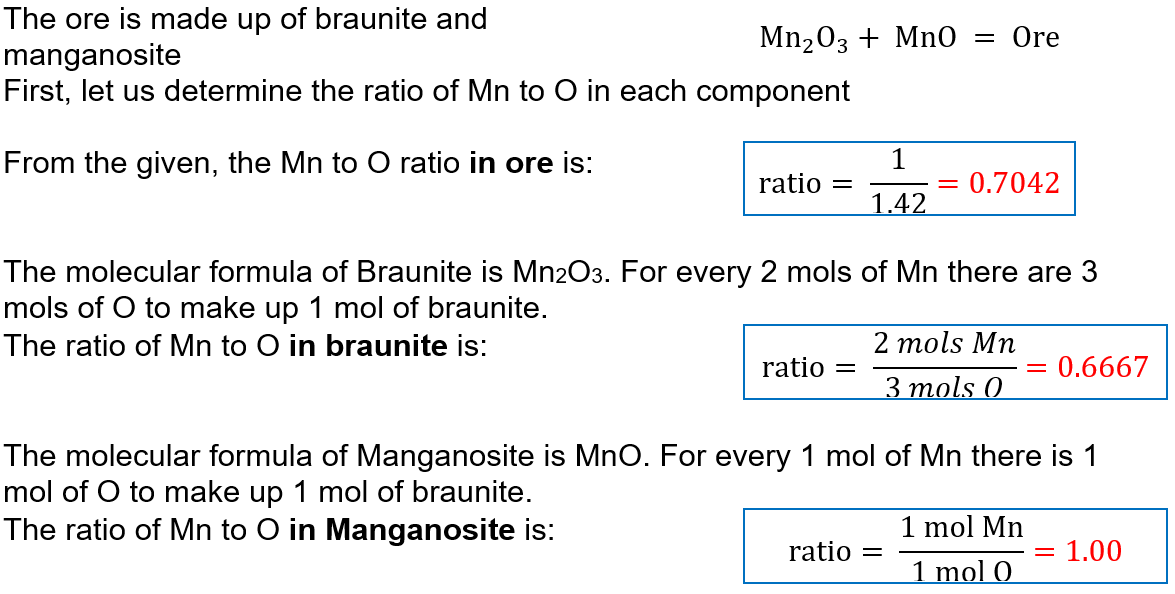# Problem: Manganese is a key component of extremely hard steel. The element occurs naturally in many oxides. A 542.3-g sample of a manganese oxide has an Mn/O ratio of 1.00/1.42 and consists of braunite (Mn2O3) and manganosite (MnO).(a) How many grams of braunite and of manganosite are in the ore?

###### FREE Expert Solution

A manganese ore is composed of braunite and manganosite. We will solve for the mass of each component in the total ore by:

1. Determining the ratio of manganese and oxygen in each compound.
2. Determining the mass ratio of Braunite to Manganosite using Mn and O ratio.
3. Solving for the mass percent of Braunite and Manganosite.
4. Solving for the mass of each component in the ore.

Step 1:85% (199 ratings)###### Problem Details

Manganese is a key component of extremely hard steel. The element occurs naturally in many oxides. A 542.3-g sample of a manganese oxide has an Mn/O ratio of 1.00/1.42 and consists of braunite (Mn2O3) and manganosite (MnO).

(a) How many grams of braunite and of manganosite are in the ore?